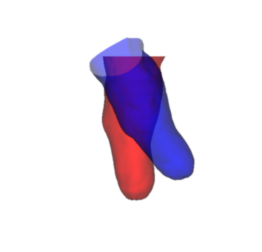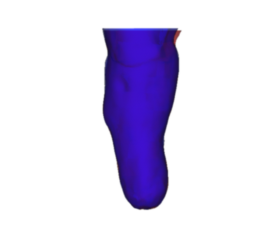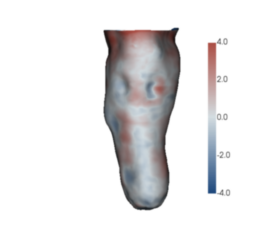# Shape comparison¶

The key operations performed by ampscan are used for comparison betweem two shapes. To do this, we need to first align the shapes.

:

from ampscan import AmpObject, align, registration

:

import warnings
warnings.filterwarnings('ignore')
%matplotlib inline

:

base = AmpObject('stl_file.stl')
move = AmpObject('stl_file_2.stl')
move.rotateAng([20, -30, 10], ang='deg')


## Alignment¶

Then we create the alignment object, although we don’t want to run the ICP algorithm yet so we set maxiter=0. By default, when you create the align object it will run the algorithm with the passed variables

:

al = align(move, base, maxiter=0)

:

import matplotlib.pyplot as plt
plt.axis('off')
im = al.genIm(crop='False')
ax = plt.imshow(im, interpolation='bicubic')As we can see, the two scans are misaliged. We can then call the ICP method on the align object and re-render

:

al.runICP()
plt.axis('off')
im = al.genIm(crop='False')
ax = plt.imshow(im, interpolation='bicubic')## Registration¶

Following alignment, we can then perform a registration stage which then enables direct shape comparison between the two shapes. First, we create the registration object, this automatically runs the registration step.

:

reg = registration(al.s, al.m, steps=10, neigh=10, smooth=1)


reg.reg is the AmpObject resulting from the registration, with the displacement field stored in the values attribute. This can then be plotted with a scalar bar

:

plt.axis('off')
reg.reg.createCMap()